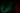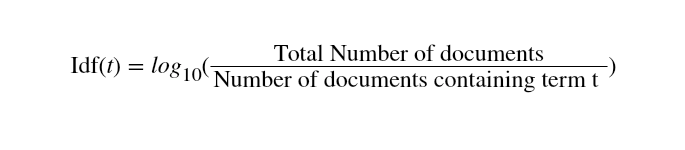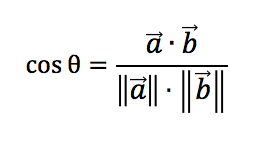# Build a Content-based recommendation engine in JS

December 19, 2019

8 mins

Machine learning has been on my radar for a long time but I have never really knuckled down and actually started learning it. That is until recently. I am a serial learner and with nothing lined up I decided to tackle some machine learning. I set myself the task of creating a recommendation engine. We interact with these ever day, through social media and online shopping as well as so many other places. I used a simple dataset from the web that consisted on 20 images with the results from a Google Vision API request. My goal was to recommend other images from the collection when a single image is selected.

I realise that Python would probably have been a better choice of language for this task but I know Javascript very well and did not want the extr burden of having to piece together the engine in a language I am not 100% comfortable with.

According to Wikipedia, a content-based recommendation engine is:

“Recommender systems or recommendation systems (sometimes replacing “system” with a synonym such as a platform or engine) are a subclass of information filtering system that seeks to predict the ‘rating’ or ‘preference’ that user would give to an item.”

Recommendation engines are active filtering systems that personalise the information coming to a user based on information known about a user. In our case, this information is the image initially selected and the data that was returned from Google Vision.

Best the end of this article we will be able to recommend a user more images based on their initial image selection.

## The pros and cons

Before we run through how. Let's talk about why. There is a reason this type of engine is so popular but there will be reasons not to use it as well.

### Pros

• Unlike other methodologies, content-based filtering does not need the data of other users, since the recommendations are specific to the user. This avoids the issue of cold starts where there is limited data
• The model captures the specific interests of the users and so can recommend niche items that may not be popular with other users

### Cons

• The model can only make recommendations based on existing interests. This limits the recommendations to known interests, stopping the broadening of the user's interests
• You are reliant on the accuracy of the labels
• Does not take into account a user's quirks. They like something but only in a very specific circumstance.

## How do content-based recommendation engines work

A content-based recommendation engine works with data that a user provides (in our case, selecting an image). Based on this data we can make suggestions to the user.

In our case, our script will progress through the following steps:

1. Training
2. Format data into a usable state
3. Calculate TF-IDF and create vectors from the formatted docs
4. Calculate similar documents
5. Use trained data to make a recommendation based on the user's image selection.

Before we start writing our recommendation engine we need to talk about a few key concepts. Namely, how are we going to decide what data to recommend?

The concepts of Term Frequency (TF) and Inverse Document Frequency (IDF) are used to determine the relative importance of a term. With that, we can use the concept of cosine similarity to determine what to recommend. We will discuss these throughout the article.

TF is simply the frequency a word appears in a document. The IDF is the frequency of a term in a whole corpus of documents. It signifies the rarity of a word and helps to boost the score of rarer terms. TD-IDF is used because it takes into account not only the isolated term but also the term within the whole corpus of documents. This model combines how important the word is in the document (local importance), with how important the word is int the whole corpus (global importance)

Cosine similarity is a metric used to determine the similarity of documents irrespective of size. Mathematically it is measuring the cosine angle between 2 vectors. In our context, the vectors will be objects containing the term as the key and the TF-IDF as the value. The value is also referred to as the vector's magnitude.

### 1. TrainingThe first step in "training" our engine is to format the data into a structure that is usable and easy to manage. The labels data that comes back from Google Cloud Vision looks something like this:

``````{
"1.jpg": [
{
"locations": [],
"properties": [],
"mid": "/m/0c9ph5",
"locale": "",
"description": "Flower",
"score": 0.9955990314483643,
"confidence": 0,
"topicality": 0.9955990314483643,
"boundingPoly": null
},
{
"locations": [],
"properties": [],
"mid": "/m/04sjm",
"locale": "",
"description": "Flowering plant",
"score": 0.9854584336280823,
"confidence": 0,
"topicality": 0.9854584336280823,
"boundingPoly": null
},
[...]
]
}``````

#### 1.a Formatting

For the purpose of this exercise, we are only concerned with the top-level key of the object (`1.jpg`) and the `description` of each of the objects in the array. But we want all of the descriptions in a single string. This will allow us to process them more easily later on.

We want the data to be in an array of objects like this:

``````const formattedData = [
{
id: "1.jpg",
content:
"flower flowering plant plant petal geraniaceae melastome family geranium wildflower geraniales perennial plant",
},
]``````

To format our data we will run it through the following function. This will return an array of all the data we need to continue training our engine. We use `Object.entries` to allow us to iterate more easily. MDN states that:

The Object.entries() method returns an array of a given object's own enumerable string-keyed property [key, value] pairs...

We then loop over the array created bt `Object.entries` plucking the necessary properties and add them to a `desc` array. Finally, we join the contents of the `desc` array and write it to the `content` property. This `formatted` array is our corpus.

``````const formatData = data => {
let formatted = []

for (const [key, labels] of Object.entries(data)) {
let tmpObj = {}
const desc = labels.map(l => {
return l.description.toLowerCase()
})

tmpObj = {
id: key,
content: desc.join(" "),
}

formatted.push(tmpObj)
}

return formatted
}``````

#### 1.b TF-IDF and Vectors

As mentioned above the TF is just the number of times a term features in a document.

For example:

``````// In the data set below the TF of plant is 3
{
id: '1.jpg',
content: 'flower flowering plant plant petal geraniaceae melastome family geranium wildflower geraniales perennial plant'
}``````

The IDF is slightly more complex to work out. The formula is:In javascript this is worked out with:

``var idf = Math.log(this.documents.length / docsWithTerm)``

We only need to above values (TF and IDF) so we can calculate the TF-IDF. It's simply TF multiplied by the IDF.

``const tdidf = tf * idf``

The next step in our process is to calculate the TF-IDF of our documents and create a vector containing the term as the key the value (vector) as the TF-IDF. We are leaning on `natural` and `vector-object` npm packages to allow us to do this easily. `tfidf.addDocument` will tokenise our `content` property. The `tfidf.listTerms` method lists our new processed documents returning an array of objects containing the TD, IDF and TD-IDF. We are only concerned with the TF-IDF though.

``````/**
* Generates the TF-IDF of each term in the document
* Create a Vector with the term as the key and the TF-IDF as the value
* @example - example vector
* {
*   flowers: 1.2345
* }
*/
const createVectorsFromDocs = processedDocs => {
const tfidf = new TfIdf();

processedDocs.forEach(processedDocument => {
});

const documentVectors = [];

for (let i = 0; i < processedDocs.length; i += 1) {
const processedDocument = processedDocs[i];
const obj = {};

const items = tfidf.listTerms(i);

for (let j = 0; j < items.length; j += 1) {
const item = items[j];
obj[item.term] = item.tfidf;
}

const documentVector = {
id: processedDocument.id,
vector: new Vector(obj)
};

documentVectors.push(documentVector);
}``````

Now we have an array of objects containing the id of the image (`1.jpg`) as the id and our vector. Our next step is to calculate the similarities between the documents.

#### 1.c Calculating similarities with Cosine similarity and the dot product

The final step in the "training" stage is to calculate the similarities between the documents. We are using the `vector-object` package again to calculate the cosine similarities. Once calculated we push them into an array that contains the image id and all the recommended images from the training. Finally, we sort them so that the item with the highest cosine similarity is first in the array.

``````/**
* Calculates the similarities between 2 vectors
* getCosineSimilarity creates the dotproduct and the
* length of the 2 vectors to calculate the cosine
* similarity
*/
const calcSimilarities = docVectors => {
// number of results that you want to return.
const MAX_SIMILAR = 20;
// min cosine similarity score that should be returned.
const MIN_SCORE = 0.2;
const data = {};

for (let i = 0; i < docVectors.length; i += 1) {
const documentVector = docVectors[i];
const { id } = documentVector;

data[id] = [];
}

for (let i = 0; i < docVectors.length; i += 1) {
for (let j = 0; j < i; j += 1) {
const idi = docVectors[i].id;
const vi = docVectors[i].vector;
const idj = docVectors[j].id;
const vj = docVectors[j].vector;
const similarity = vi.getCosineSimilarity(vj);

if (similarity > MIN_SCORE) {
data[idi].push({ id: idj, score: similarity });
data[idj].push({ id: idi, score: similarity });
}
}
}

// finally sort the similar documents by descending order
Object.keys(data).forEach(id => {
data[id].sort((a, b) => b.score - a.score);

if (data[id].length > MAX_SIMILAR) {
data[id] = data[id].slice(0, MAX_SIMILAR);
}
});

return data;``````

Under the hood, the `getCosineSimilarity` method is doing a number of things.

It generates the dot product, this operation takes 2 vectors and returns a single (scalar) number. It is a simple multiplication of each component in both vectors added together.

``````a = [1.7836, 3]
b = [4, 0.5945]

a.b = 1.7836 * 4 + 3 *0.5945 = 8.9176``````

With the dot product calculated we just need to reduce the vector values of each document down to scalar values. This is done by taking the square root of each value multiplied by itself added together. The `getLength` method below is doing this calculation.

``````const getLength = () => {
let l = 0

this.getComponents().forEach(k => {
l += this.vector[k] * this.vector[k]
})

return Math.sqrt(l)
}``````

The actual cosine similarity formula looks like this:and in javascript looks like this:

``````const getCosineSimilarity = vector => {
return this.getDotProduct(vector) / (this.getLength() * vector.getLength())
}``````

The training is complete!!## 2. Getting our recommendations

Now we have completed the training phase we can simply request the recommended images from the training data.

``````const getSimilarDocuments = (id, trainedData) => {
let similarDocuments = trainedData[id]

if (similarDocuments === undefined) {
return []
}

return similarDocuments
}``````

This will return an array of objects containing the recommended images and their cosine similarity score.

``````// e.g
;[
{ id: "14.jpg", score: 0.341705472305971 },
{ id: "9.jpg", score: 0.3092133517794513 },
{ id: "1.jpg", score: 0.3075994367748345 },
]``````

## Wrap up

I hope you were able to follow along. I learned so much from this exercise and it has really piqued my interest in machine learning.